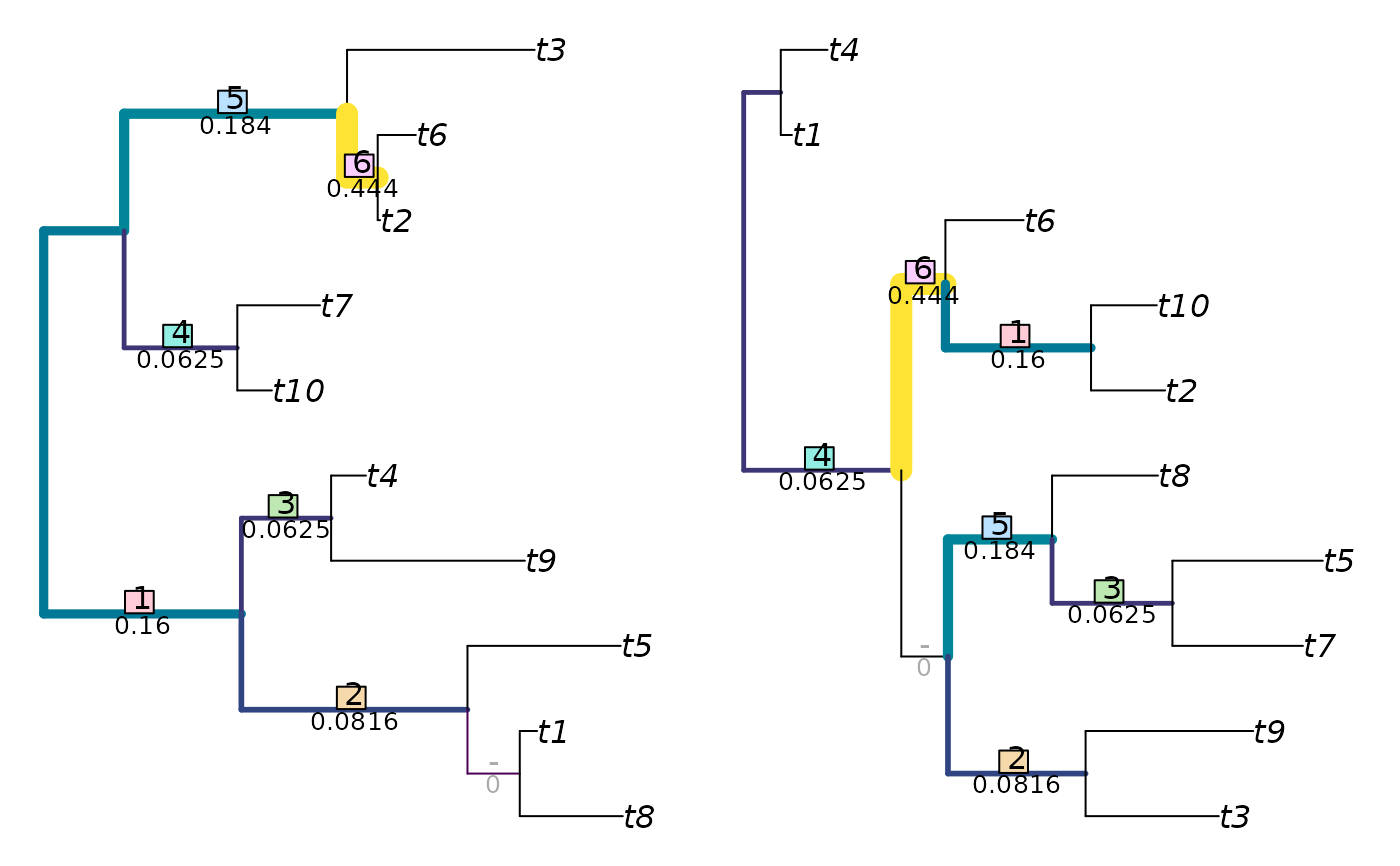JaccardRobinsonFoulds(
tree1,
tree2 = NULL,
k = 1L,
allowConflict = TRUE,
similarity = FALSE,
normalize = FALSE,
reportMatching = FALSE
)

JaccardSplitSimilarity(
splits1,
splits2,
nTip = attr(splits1, "nTip"),
k = 1L,
allowConflict = TRUE,
reportMatching = FALSE
)

## Arguments

tree1

Trees of class phylo, with leaves labelled identically, or lists of such trees to undergo pairwise comparison. Where implemented, tree2 = NULL will compute distances between each pair of trees in the list tree1 using a fast algorithm based on Day (1985).

tree2

Trees of class phylo, with leaves labelled identically, or lists of such trees to undergo pairwise comparison. Where implemented, tree2 = NULL will compute distances between each pair of trees in the list tree1 using a fast algorithm based on Day (1985).

k

An arbitrary exponent to which to raise the Jaccard index. Integer values greater than one are anticipated by Böcker et al. The Nye et al. metric uses k = 1. As k increases towards infinity, the metric converges to the Robinson–Foulds metric.

allowConflict

Logical specifying whether to allow conflicting splits to be paired. If FALSE, such pairings will be allocated a similarity score of zero.

similarity

Logical specifying whether to report the result as a tree similarity, rather than a difference.

normalize

If a numeric value is provided, this will be used as a maximum value against which to rescale results. If TRUE, results will be rescaled against a maximum value calculated from the specified tree sizes and topology, as specified in the 'Normalization' section below. If FALSE, results will not be rescaled.

reportMatching

Logical specifying whether to return the clade matchings as an attribute of the score.

splits1

Logical matrices where each row corresponds to a leaf, either listed in the same order or bearing identical names (in any sequence), and each column corresponds to a split, such that each leaf is identified as a member of the ingroup (TRUE) or outgroup (FALSE) of the respective split.

splits2

Logical matrices where each row corresponds to a leaf, either listed in the same order or bearing identical names (in any sequence), and each column corresponds to a split, such that each leaf is identified as a member of the ingroup (TRUE) or outgroup (FALSE) of the respective split.

nTip

(Optional) Integer specifying the number of leaves in each split.

## Value

JaccardRobinsonFoulds() returns an array of numerics providing the distances between each pair of trees in tree1 and tree2, or splits1 and splits2.

## Details

In short, the Jaccard–Robinson–Foulds metric is a generalized Robinson-Foulds metric: it finds the optimal matching that pairs each split in one tree with a similar split in the second. Matchings are scored according to the size of the largest split that is consistent with both of them, normalized against the Jaccard index, and raised to an arbitrary exponent. A more detailed explanation is provided in the vignettes.

By default, conflicting splits may be paired.

Note that the settings k = 1, allowConflict = TRUE, similarity = TRUE give the similarity metric of Nye et al. (2006); a slightly faster implementation of this metric is available as NyeSimilarity().

The examples section below details how to visualize matchings with non-default parameter values.

## Normalization

If normalize = TRUE, then results will be rescaled from zero to one by dividing by the maximum possible value for trees of the given topologies, which is equal to the sum of the number of splits in each tree. You may wish to normalize instead against the maximum number of splits present in a pair of trees with n leaves, by specifying normalize = n - 3.

Other tree distances: KendallColijn(), MASTSize(), MatchingSplitDistance(), NNIDist(), NyeSimilarity(), PathDist(), Robinson-Foulds, SPRDist(), TreeDistance()

## Examples

set.seed(2)
tree1 <- ape::rtree(10)
tree2 <- ape::rtree(10)
JaccardRobinsonFoulds(tree1, tree2, k = 2, allowConflict = FALSE)
#>  12.0105
JaccardRobinsonFoulds(tree1, tree2, k = 2, allowConflict = TRUE)
#>  11.40222

JRF2 <- function (tree1, tree2, ...)
JaccardRobinsonFoulds(tree1, tree2, k = 2, allowConflict = FALSE, ...)

VisualizeMatching(JRF2, tree1, tree2, matchZeros = FALSE)